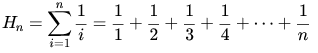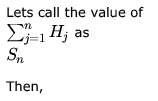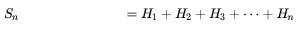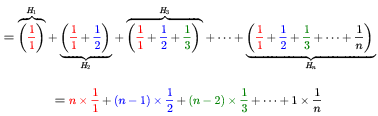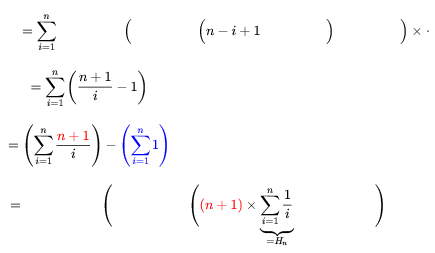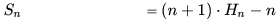Courses

# Test: Combinatory- 2

## 25 Questions MCQ Test RRB JE for Computer Science Engineering | Test: Combinatory- 2

Description
This mock test of Test: Combinatory- 2 for Computer Science Engineering (CSE) helps you for every Computer Science Engineering (CSE) entrance exam. This contains 25 Multiple Choice Questions for Computer Science Engineering (CSE) Test: Combinatory- 2 (mcq) to study with solutions a complete question bank. The solved questions answers in this Test: Combinatory- 2 quiz give you a good mix of easy questions and tough questions. Computer Science Engineering (CSE) students definitely take this Test: Combinatory- 2 exercise for a better result in the exam. You can find other Test: Combinatory- 2 extra questions, long questions & short questions for Computer Science Engineering (CSE) on EduRev as well by searching above.
QUESTION: 1

### In how many ways can three person, each throwing a single die once, make a score of 11

Solution:

The sum 11 can be broken as 6 + 5
Let us name the players as A, B and C.
Case 1: Fix 6 and break 5

Suppose A throws 6. Players B and C can throw dice in following four ways to make sum 5
(1,4), (2,3), (3,2) and (4,1)
As any of the three players can throw value 6, so there are total 3 x 4 = 12

Case 2: Fix 5 and break 6

Suppose A throws 5. Players B and C can throw dice in following five ways to make sum 6
(1,5), (2,4), (3,3), (4,2), (5,1)
As any of the three players can throw value 5, so there are total 3 x 5 = 15
Adding case 1 and case 2 there are total  12 + 15 = 27 ways

QUESTION: 2

### Match the pairs in the following questions by writing the corresponding letters only.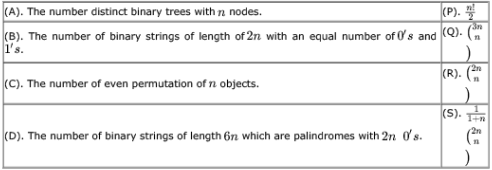Solution:

(A) - S Catalyn no
(B) - R. Choosing n locations out of 2n to place 0. Remaining automatically become 1.
(C) -P An even permutation is a permutation obtainable from an even number of two-element swaps, For a set of elements and n > 2, there are  n! / 2 even permutations.
(D) -> Q
Length = 6n, as it is palindrome, we need to only consider half part.
Total Length to consider 3n (Remaining 3n will be revese of this 3n)
now Choosing n 0's out of 3n. So Q is correct for D.

QUESTION: 3

### It is required to divide the  members of a club into  disjoint teams of 2 members each. The teams are not labelled. The number of ways in which this can be done is:

Solution:

2n member to be n teams with 2 member each and teams are unordered so we can exchange n team member among them.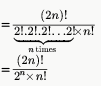QUESTION: 4

In how many ways can the letters of the word ABACUS be rearranged such that the vowels always appear together?

Solution:

Take AAU together and treat it like 1 entity. Now arrange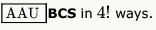Then, the AAU can be arranged in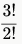ways because A has been repeated twice.
So, total arrangements =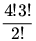QUESTION: 5

How many sub strings of different lengths (non-zero) can be found formed from a character string of length n ?

Solution:

assuming an string of length n provided all alphabets are distinct..
no of strings of length 1 = n
no of strings of length 2 = n-1
no of strings of length 3 = n-2 .
.
.
no of string of length n = 1
total = n + (n -1) + (n - 2) + (n - 2) + ..... + 1

= n(n+1)/2

QUESTION: 6

Find the number of binary strings w of length 2n with an equal number of  1's and 0's  and the property that every prefix of w has at least as many  0's as 1's.

Solution:

Answer to a is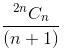which is the Catalan number.
This is also equal to the number of possible combinations of balanced parenthesizes.

QUESTION: 7

How many 4-digit even numbers have all 4 digits distinct

Solution:
• If the number ends with a 0 then there are 9 choices for the first digit, 8 for the second and 7 for the third, which makes 1×9×8×7=504 possibilities.
• If the number is even ending with something else than 0 then there are 4 choices for the last digit, 8 choices for the first digit (no 0 nor the last digit), 8 for the second digit and 7 for the third digit, which makes 4×8×8×7=1792

​Together, this gives 2296 numbers with 4 distinct digits that are even. Note that this does not allow leading 0, as you see to want it based from the question

QUESTION: 8

Let A be a sequence of 8 distinct integers sorted in ascending order. How many distinct pairs of sequences, B and C are there such that

i. each is sorted in ascending order,
ii. B has 5 and C has 3 elements, and
iii. the result of merging B and C gives A

Solution:

select any 3 elements from given 8 elements - 8C3

QUESTION: 9

n couples are invited to a party with the condition that every husband should be accompanied by his wife. However, a wifeneed not be accompanied by her husband. The number of different gatherings possible at the party is

Solution:

Possible outcome for a couple:

1. only wife comes
2. both come
3. none come

Thus 3 possibilities for each couple, so 3 x 3 x 3 x ... n times = 3n

QUESTION: 10

m identical balls are to be placed in n distinct bags. You are given that m > kn, where  is a natural number > 1. In howmany ways can the balls be placed in the bags if each bag must contain at least  balls?

Solution:

As there have to be atleast k balls in each bag, so firstly put k balls in each bag i.e k*n balls.
Then now we have total m-k*n balls remaining.
We can use balls & sticks method now !
n bags= n variables, they need to equal to m-k*n, no restrictions on how many balls in each bag !
x1 + x2 + ... + xn = m- k*n , x1,x2..xn >=0.
So when we solve it
We get
C(m - k*n + n - 1, n-1 ) = C(m - k*n + n - 1, m- k*n )

QUESTION: 11

Mala has the colouring book in which each English letter is drawn two times. She wants to paint each of these 52 prints with one of k colours, such that the colour pairs used to colour any two letters are different. Both prints of a letter can also be coloured with the same colour. What is the minimum value of k that satisfies this requirement?

Solution:

This question is slightly ambigous. So first let us understand what question is asking. So in a book, we have letters A-Z and each letter is printed twice, so there are 52 letters. Now we have to color each letter, so we need a pair of colors for that, because each letter is printed twice. Also in a pair, both colors can be same. Now condition is that a pair of colors can't be used more than once.
So suppose Mala has 3 colors : Red, Blue, Green. She can color as follows : 1:(Red,Red),  2:(Blue,Blue), 3:(Green,Green), 4: (Red,Blue), 5: (Red,Green),
6 : (Blue,Green).
Now we don't have more pairs of colors left, we have used all pairs, but could color only 6 letters out of 26. So question is to find minimum no. of colors, so that we could color all 26 letters.
So if Mala has k colors, she can have k pairs of same colors, thus coloring k letters, then kC2 other pairs of colors, thus coloring kC2 more letters.

So total no. of letters colored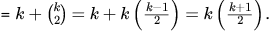So we want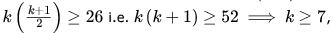so option (C) is correct.

QUESTION: 12

Suppose that a robot is placed on the Cartesian plane. At each step it is allowed to move either one unit up or one unit right, i.e., if it is at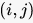then it can move to either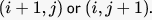How many distinct paths are there for the robot to reach the point (10,10) starting from the initial position (0,0)?

Solution:

Say,  r = Move Right and
u = Move Up
so using 10 combination of r and 10 combinations of u moves we get a solution.

Convert the graphical moves to text and one such solution we get =
{u, u, u, u, u, u, u, u, u, u, r, r, r, r, r, r, r, r, r, r} now all possible arrangements of them is given by =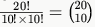QUESTION: 13

The number of binary strings of  zeros and  ones in which no two ones are adjacent is

Solution:

first place n zeroes side by side _ 0 _ 0 _ 0 ... 0 _
k 1's can be placed in any of the (n+1) available gaps hence number of ways  = n+1Ck

QUESTION: 14

Two girls have picked 10 roses, 15 sunflowers and 15 daffodils. What is the number of ways they can divide the flowers among themselves?

Solution:

number of ways roses can be distributed - {(0, 10), (1, 9), (2, 8).....(10, 0)} - 11 ways
similarly sunflowers and daffodils can be distributed in 16 ways each
total number of ways 11 x 16 x 16 = 2816

*Answer can only contain numeric values
QUESTION: 15

A pennant is a sequence of numbers, each number being 1 or 2. An -pennant is a sequence of numbers with sum equal to n For example, (1,1,2) is a 4-pennant. The set of all possible 1-pennants is (1), the set of all possible 2-pennants is (2), (1,1) and the set of all 3-pennants is (2,1), (1,1,1,), (1,2). Note that the pennant (1,2) is not the same as thepennant (2,1). The number of 10-pennants is________

Solution:

Let we denote number of n-pennants by f(n), so f(10) is number of 10-pennants.

So all 10-pennants can be formed by 8-pennants and 9-pennants, and no other pennant (since we can add only 1 or 2 into a sequence)
So f(10) = f(9) + f(8)
This is in fact a fibonacci sequence, in which F(1) = 1, f(2) = 2, so this sequence becomes
1, 2, 3, 5, 8, 13, 21, 34, 55, 89,..
So f(10) = 89.

Numbers could be any one of
(1,1,1,1,1,1,1,1,1,1),(1,1,1,1,1,1,1,1,2),(1,1,1,1,1,1,2,2),(1,1,1,1,2,2,2),(1,1,2,2,2,2),(2,2,2,2,2)
So, the number of 10 penants =1+  9!/8! + 8!/6!2!  + 7!/4!3! + 6!/2!4! +1 =89

*Answer can only contain numeric values
QUESTION: 16

The number of 4 digit numbers having their digits in non-decreasing order (from left to right) constructed by using the digits belonging to the set {1, 2, 3} is ________.

Solution:

Dynamic programming Approach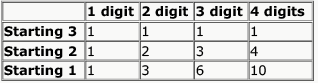Here Starting 1 means numbers starting with 1. And cellis for number of numbers starting with  and having  digits.
We can have the relation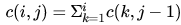as per the non-decreasing condition given in the question. So, our answer will be c(1, 4) + c(2, 4) + c(3, 4) = 1 + 4 + 10 = 15

Brute force
3 3 3 3
2 2 2 2
2 2 2 3
2 2 3 3
2 3 3 3
1 1 1 1
1 1 1 2
1 1 1 3
1 1 2 2
1 1 2 3
1 1 3 3
1 2 2 2
1 2 2 3
1 2 3 3
1 3 3 3

you can arrive at a solution by constructing a graph for each starting digit. for example root 3 means - starting with 3 it can have 3 child 1,2,3 and the construction goes
3 can three children 1, 2,3
2 can have two children 1, 2
1 can have only 1 as child. Graph need to be done till three levels and finally count total number of leaves

QUESTION: 17

The number of substrings (of all lengths inclusive) that can be formed from a character string of length n is

Solution:

no. of substrings of length n is 1
no. of substrings of length n-1 is 2
no. of substrings of length n-2 is 3
so n(n+1)/2

QUESTION: 18

In how many ways can b blue balls and r red balls be distributed in n distinct boxes?

Solution:

r red balls can be distributed into n distinct boxes in C(n+r-1,r) = .(n+r-1)! / (n-1)! r!
b blue balls can be distributed in C(n+b-1,b) = (n+b-1)! / (n-1)! b!
By product rule total ways are (n+b-1)! (n+r-1)! / (n-1)! b! (n-1)! r!

QUESTION: 19

In how many ways can we distribute 5 distinct balls, B1, B2, ..., B5 in 5 distinct cells, C1, C2, ...., C5 such that Ball Bi is not in cell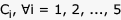and each cell contains exactly one ball?

Solution:

Use Derangement concept D5= 44

QUESTION: 20

A line L in a circuit is said to have a stuck-at-0 fault if the line permanently has a logic value 0. Similarly a line L in a circuit is said to have a stuck-at-1 fault if the line permanently has a logic value 1. A circuit is said to have a multiple stuck-at fault if one or more lines have stuck at faults. The total number of distinct multiple stuck-at faults possible in a circuit with N lines is

Solution:

This is because the total possible combinations (i.e a line may either be at fault (in 2 ways i.e stuck at fault 0 or 1) or it may not be , so there are only 3 possibilities for a line) is 3N. In only one combination the circuit will have all lines to be correct (i.e not at fault.) Hence 3N-1. (as it has been said that circuit is said to have multiple stuck up fault if one or more line is at fault)

QUESTION: 21

Consider the recurrence relation a1 = 8, an = 6n2 + 2n + an-1. Let a99 = K x 104  . The value of K is __________.

Solution:

an = 6n2 + 2n + an−1
= 6n2 + 2n + 6(n − 1)2 + 2(n − 1) + an−2
= 6n2 + 2n + 6(n − 1)2 + 2(n − 1) + 6(n − 2)2 + 2(n − 2)+. . . . . . +a1
= 6n2 + 2n + 6(n − 1)2 + 2(n − 1) + 6(n − 2)2 + 2(n − 2)+. . . . . . +6.12 + 2.1
= 6(n2 + (n − 1)2 +. . . +22 + 12) + 2(n + (n − 1)+. . . +2 + 1)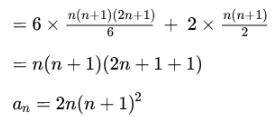for n = 99 a99 = 2 x 99 x (99+1)= 198 x 104

QUESTION: 22

Let an be the number of -bit strings that do NOT contain two consecutive 1s. Which one of the following is the recurrence relation for an?

Solution: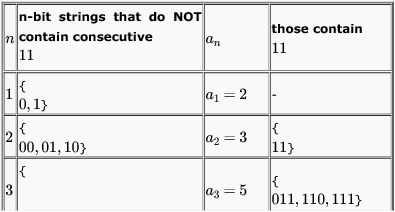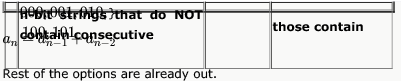Alternatively, we can get a string in  an by appending "0" to any string in  an-1 as well as by appending "01" to any string in an-2 and the two cases are mutually exclusive (no common strings) as well as exhaustive (covers all cases).

QUESTION: 23

Consider the sequence S0,S1,S2,.... defined as follows: S0 = 0, S1 = 1 and Sn =  2Sn-1 + Sn-2 for n > 2. Which of the following statements is FALSE?

Solution:

Sn = 2Sn−1 + Sn−2

Characterstic polynomial for this recurrence is x2 = 2x + 1

x2 − 2x − 1 = 0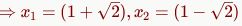The solution to the recurrence relation is of the form  :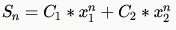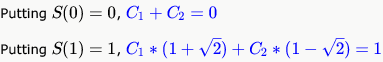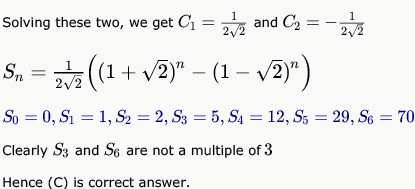*Multiple options can be correct
QUESTION: 24

Consider the sequence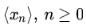defined by the recurrence relation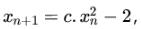where c >0.
Suppose there exists a non-empty, open interval (a,b) such that for all  satisfying  a < x0 < b , the sequence converges to a limit. The sequence converges to the value?

Solution:

Since it converges, we can write:
x = cx2 − 2
or
cx2 − x − 2 = 0
Solving for x: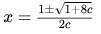So both A) and B) can be the values.

QUESTION: 25

Let H1, H2, H3, ... be harmonic numbers. Then, for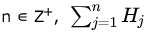can be expressed as

Solution:

The  nthHarmonic Number  is defined as the summation of the reciprocals of all numbers from  to .